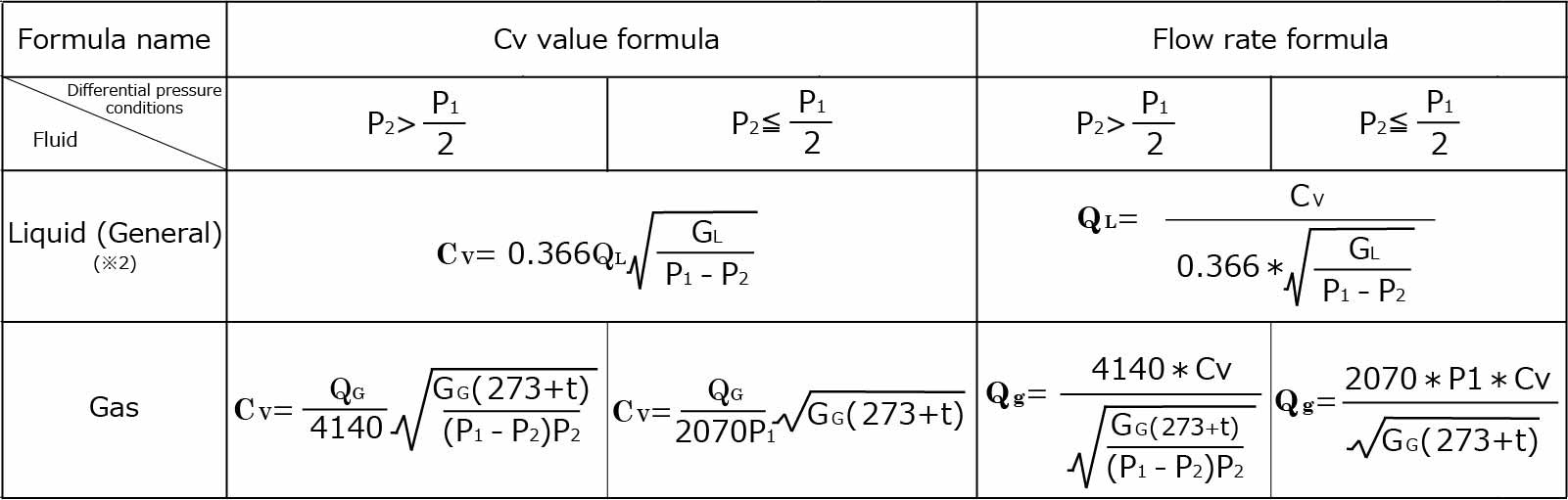Product Information

# Cv Value & Flow Rate Calculator

## What is Cv value?

The valve flow coefficient according to the JIS standard, represents the flow capacity in US gallons/minute of 60° F pure water when it is flowing through the valve with a pressure difference of 1 psi at the specified travel (operation range).

## Cv value and flow rate calculation

Calculating the flow rate from the Cv value requires complex calculations. We have created a Cv value and flow rate calculator, so please utilize it.

1 Select
calculation method
2 Select fluid type
Enter specific gravity
3 Enter flow rate
Select unit
4 Select pressure
With both gas and liquid vacuum (negative pressure), enter the absolute pressure
Select pressure unit
Enter pressure
Differential pressure
Reference Cv value00.00
※ Depends on the following formulas.【Explanation of symbols】
QL [m3/h] : Liquid flow rate（h : hours）
Qg [m3/h (normal) ] : Gas flow rate in standard conditions (15℃ 0.1013 MPa abs)
P1 [MPa abs] : Primary absolute pressure ※3
P2 [MPa abs] : Secondary absolute pressure ※3
t [℃] : Fluid temperature
GL : Liquid relative density（Water = 1）
Gg : Gas relative density（Air = 1）
【Notes on use】
※1 Please handle the calculation result as reference value. It is a standard for selection of valves, depending on the specific piping conditions and conditions of use, there is a possibility that actual results may differ from calculation results.
※2 Calculation of viscosity correction is necessary when the fluid is liquid and the kinematic viscosity is 20 mPa・s or more and the calculated Cv value is 0.01 or less. Please contact us if the fluid specification requires viscosity correction.
※3 For P1 and P2, please use pressure close to the valve. If calculation is made using the pressure at the point away from the valve, large errors may occur in the calculation results due to pressure loss etc. of piping.